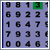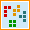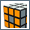Exercise Your Brain -> Interactive Brain Games -> Math Search GameMath Search:

Solve the equation and search for the answer.

This is a traditional word search game with a mathematical twist. Instead of searching for words, you search for numbers representing the answers to an equation.

You need to be able to calculate the answer to the equation and then search the answer in a grid of numbers.

### Keep on exercising your mind:Play More Brain Games These games require apt thinking rather than quick reflexes.Back to Puzzles, Riddles & Brainteasers Think you’re smart enough?